Next: The Potential Derivative Up: Writing Down the Equations Previous: Variable Rescalings

### The Program Potential

The starting point for all the equations you need to enter in the program is the potential for your model, which in turn defines your field content. So the first expression you need to derive in program variables is the potential itself. The program potential is defined as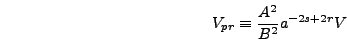(4.8)

whereis the physical potential of your model, expressed in terms of program fields. This is easiest to illustrate with an example, so continuing with the TWOFLDLAMBDA model we have(4.9)

so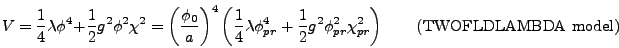(4.10)

and(4.11)

Because the coupling constantsand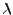appear in the program potential only in the combination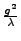we choose for convenience to define a new variable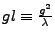.Next: The Potential Derivative Up: Writing Down the Equations Previous: Variable Rescalings